Function Repository Resource:

# TransfiniteInterpolation

Transfinite interpolation of functions representing boundary curves of a surface

Contributed by: Jan Mangaldan
 ResourceFunction["TransfiniteInterpolation"][{fs,fn},{fw,fe},{x,xmin,xmax},{y,ymin,ymax}] gives the transfinite interpolation of the functions fw and fe over x and the functions fs and fn over y.

## Details

In two-dimensional transfinite interpolation, an interpolating function f[x,y] is constructed such that the boundary conditions f[x,ymin]=fs[x], f[x,ymax]=fn[x], f[xmin,y]=fw[y] and f[xmax,y]=fe[y] are satisfied. In effect the result fills in the surface from specified boundary curves.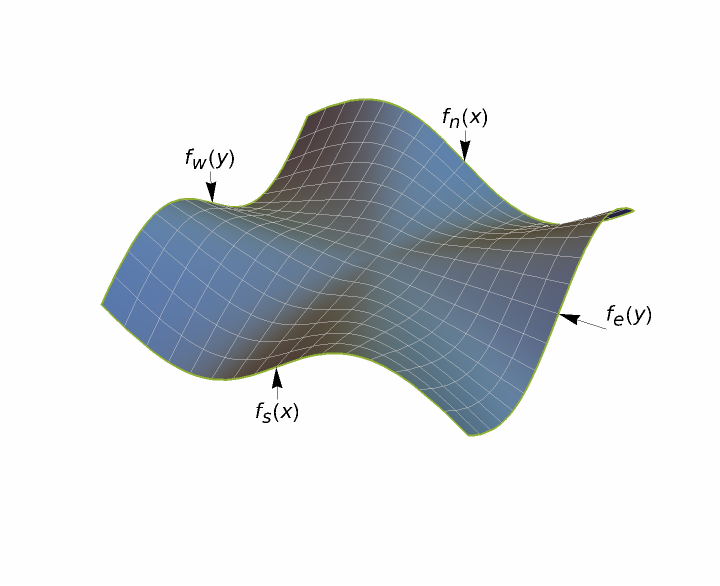## Examples

### Basic Examples (2)

Build the transfinite interpolant of a given set of slice functions:

 In:=Out=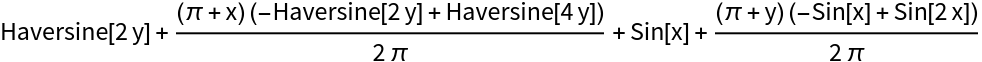Plot the transfinite interpolant:

 In:=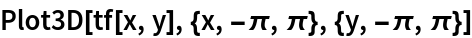Out=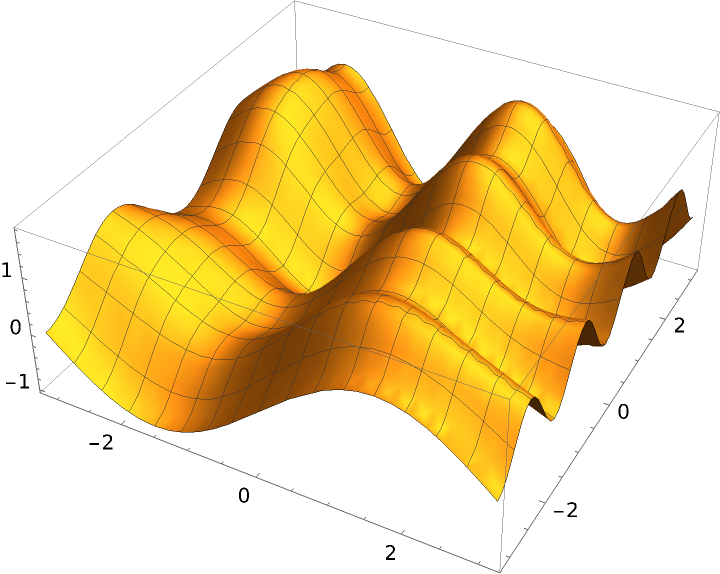### Properties and Relations (4)

Construct a transfinite interpolant:

 In:=Out=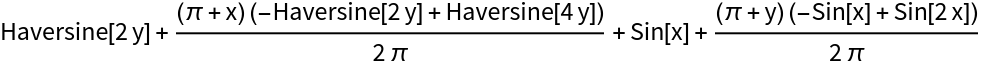Construct another interpolant by solving a Laplace equation:

 In:=Out=Compare the two interpolating functions:

 In:=Out=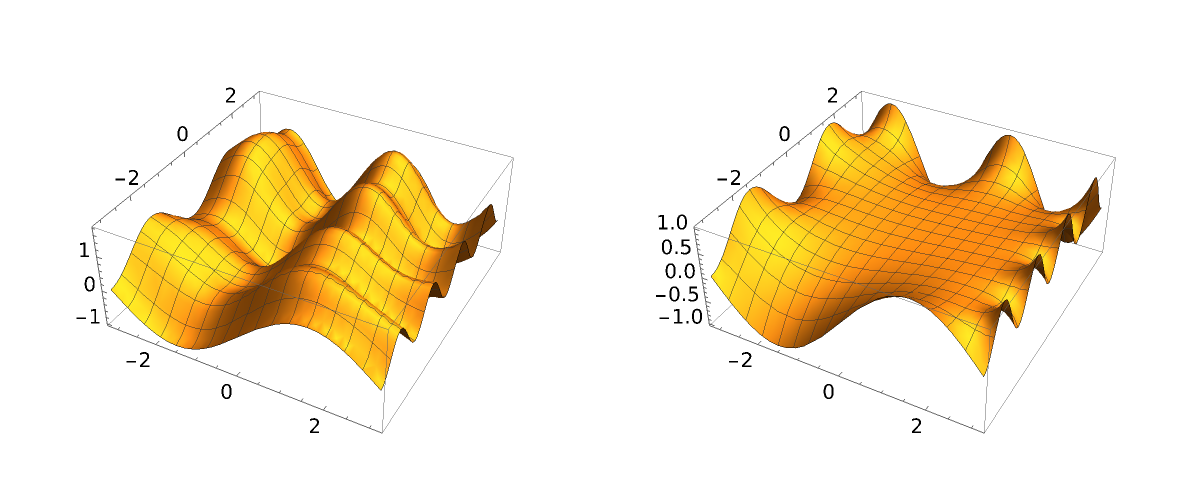The two functions agree at the boundaries, but behave differently within them:

 In:=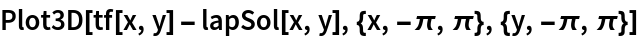Out=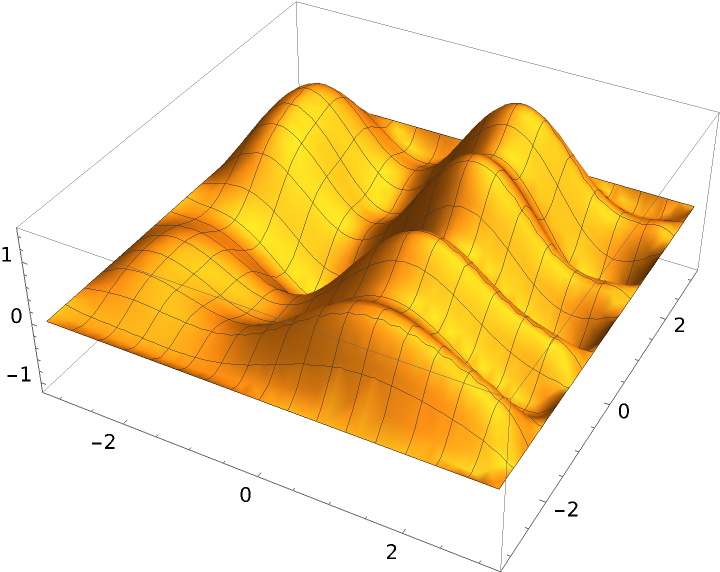## Version History

• 1.0.0 – 27 May 2022